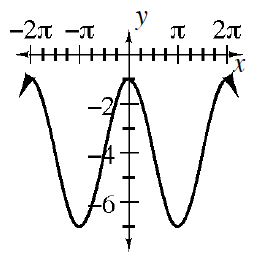Home > INT3 > Chapter 11 > Lesson 11.2.4 > Problem11-111

11-111.
1. Consider the function f(x) = 3 sin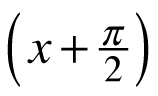– 4. Homework Help ✎

1. How is its graph different from the graph of g(x) = sin(x)?

2. Sketch the graph of y = f(x).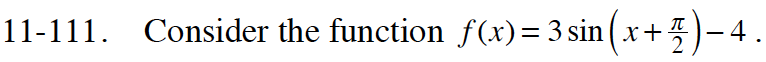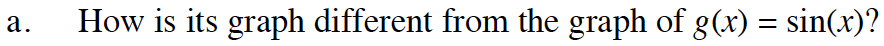Look at the function's equation. What parts are different from the equation for g(x) = sin(x)?
How is the graph shifted horizontally? Vertically? How is it stretched?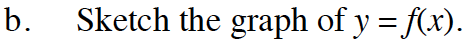Begin by drawing an axis that reflects the vertical shift. In this case it is y = −4.